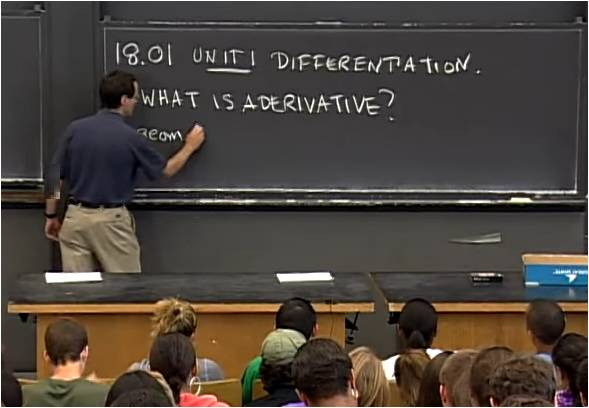# InfoCoBuild

## 18.01 Single Variable Calculus

18.01 Single Variable Calculus (Fall 2006, MIT OCW). This consists of 39 video lectures given by Professor David Jerison, on single variable calculus. This is an introductory calculus course covering differentiation and integration of functions of one variable, with applications: differentiation, application of differentiation, definite integral and its applications, techniques of integration, and a brief discussion of infinite series. (from ocw.mit.edu)Lecture 01 - Derivatives, Slope, Velocity, Rate of Change Lecture 02 - Limits, Continuity Lecture 03 - Derivatives Lecture 04 - Chain Rule Lecture 05 - Implicit Differentiation Lecture 06 - Exponential and Log Lecture 07 - Hyperbolic Functions Lecture 08 - No Class; Exam Lecture 09 - Linear and Quadratic Approximations Lecture 10 - Curve Sketching Lecture 11 - Max-min Problems Lecture 12 - Related Rates Lecture 13 - Newton's Method and Other Applications Lecture 14 - Mean Value Theorem Lecture 15 - Differentials, Antiderivatives Lecture 16 - Differential Equations Lecture 17 - No Class; Exam Lecture 18 - Definite Integrals Lecture 19 - First Fundamental Theorem Lecture 20 - Second Fundamental Theorem Lecture 21 - Applications to Logarithms and Geometry Lecture 22 - Volumes by Disks and Shells Lecture 23 - Work, Average Value, Probability Lecture 24 - Numerical Integration Lecture 25 - Exam 3 Review Lecture 26 - No Class; Exam Lecture 27 - Trigonometric Integrals and Substitution Lecture 28 - Integration by Inverse Substitution Lecture 29 - Partial Fractions Lecture 30 - Integration by Parts Lecture 31 - Parametric Equations Lecture 32 - Polar Coordinates Lecture 33 - Exam 4 Review Lecture 34 - No Class; Exam Lecture 35 - Indeterminate Forms Lecture 36 - Improper Integrals Lecture 37 - Infinite Series Lecture 38 - Taylor's Series Lecture 39 - Final Review

 References 18.01 Single Variable Calculus Instructors: Prof. David Jerison. Lecture Notes. Exams and Solutions. Subtitles/Transcript. Assignments (no Solutions). This covers differentiation and integration of functions of one variable, with applications.